# RF Passive Differential Probe

Électronique - 10-03-2023

## Presentation

This passive RF differential probe is a cost-effective solution for in-circuit measurements of RF systems operating within the frequency range of 500 MHz to 6 GHz. It is well-suited for situations where a test port is unavailable and it is engineered to have minimal impact on the device under test.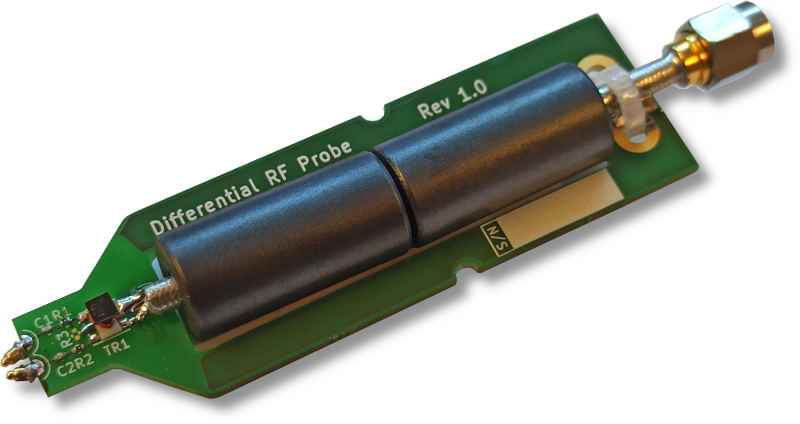Rev 1.0 of the RF probe.

The probe's spring-loaded pins can be positioned at any angle to fit the ground-signal track spacing, with a practical lower bound of 1 mm, equivalent to the length of an 0402 component.

Compared to commercial active probes, this probe is significantly more affordable and has a different input power range, making it suitable for use on high-power RF circuits for instance.

A typical application for this probe is for tuning multiple-stage RF power amplifiers.

The total bill of materials (BOM) cost is under \$15 per unit.

I am grateful to PTS GmbH for their support and resources during the development of this project, as well as for allowing me the freedom to continue working on it independently.

## Performance

This table lists the key characteristics of the probe:

Parameter Typical value
Usable frequency range * 500 MHz to 6 GHz
Attenuation at 2 GHz ** -22.5 dB
Maximum input power > 44 dBm
Flatness over 100 MHz < 1.5 dB
Loss for the DUT ** < 0.5 dB
DC protection 50 Vdc

Notes:

• (*) The measurements documented in this article only go up to 3.2 GHz due to the available test equipement.
• (**) These values depend on the value of series resistors. The value given in this table are for the baseline value of 240 Ω. See the sections Principle of operation and Simulation below for an analysis of the effect of this parameter on the probe's performance.

This probe is designed to be used with a Vector Network Analyzer (VNA), Spectrum Analyzer, or Oscilloscope. To use it effectively, it is important to obtain the correction curve for the specific probe to be able to take quantitative power measurements.

For instance, the typical recommended usage with a VNA is to first do a through calibration of the device with a reference terminated test track, and then probe RF lines as needed.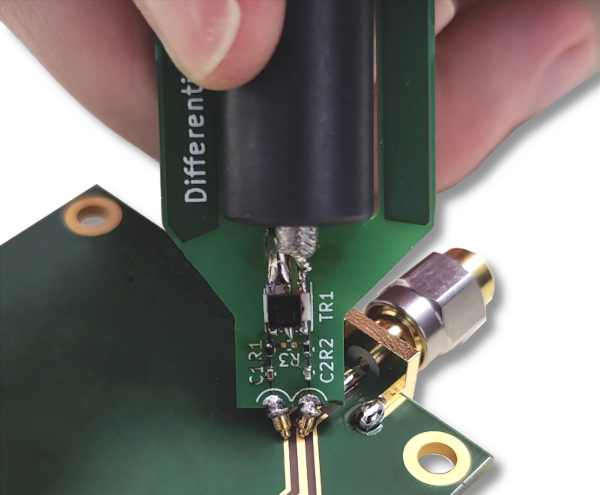Probing a PCB microstrip transmission line.

All the files are in the GitHub repository: https://github.com/CGrassin/rf_differential_probe

Note: when using it with a oscilloscope, a 50 Ω termination is required (either internal for devices that have this feature, or externally as close to the input as possible).

## Technical details

### Principle of operation

This probe has a simple construction, as shown in the diagram below: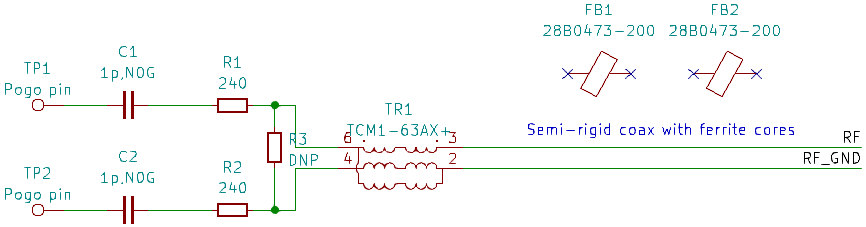The RF probe circuit diagram in KiCad.

This is the principle of operation:

• TP1 and TP2 are spring-loaded probing pins that should be as short as possible.
• C1 and C2 block DC to protect the transformer (maximum 30 mA).
• R1 and R2 limit the power that goes through the probe, thereby setting its attenuation and load on the Device Under Test (DUT). They should have the same value, R1=R2=R.
• FB1 and FB2 are ferrite beads over a piece of coaxial cable and act as a common-mode choke to suppress low-frequency common-mode current from flowing on the outside of the coax shield.

The theoretical attenuation of R1 and R2 follows this equation (ignoring losses in the transformer and transmission line):This is a plot of the theoretical attenuation for values of R from 50 Ω to 1000 Ω: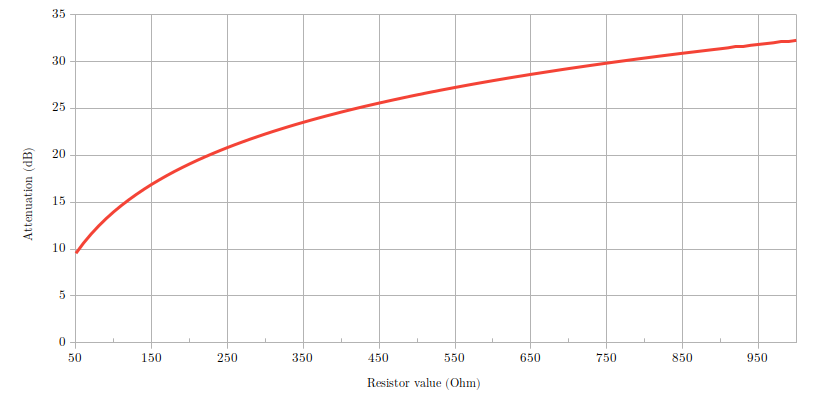### Simulation model

I created an S-parameter simulation for this probe using QucStudio. The simulation uses the S-parameter file provided by Mini-Circuits for the transformer, and lumped component models for everything else. Transmission line losses were not taken into account.

Here's the simulation model: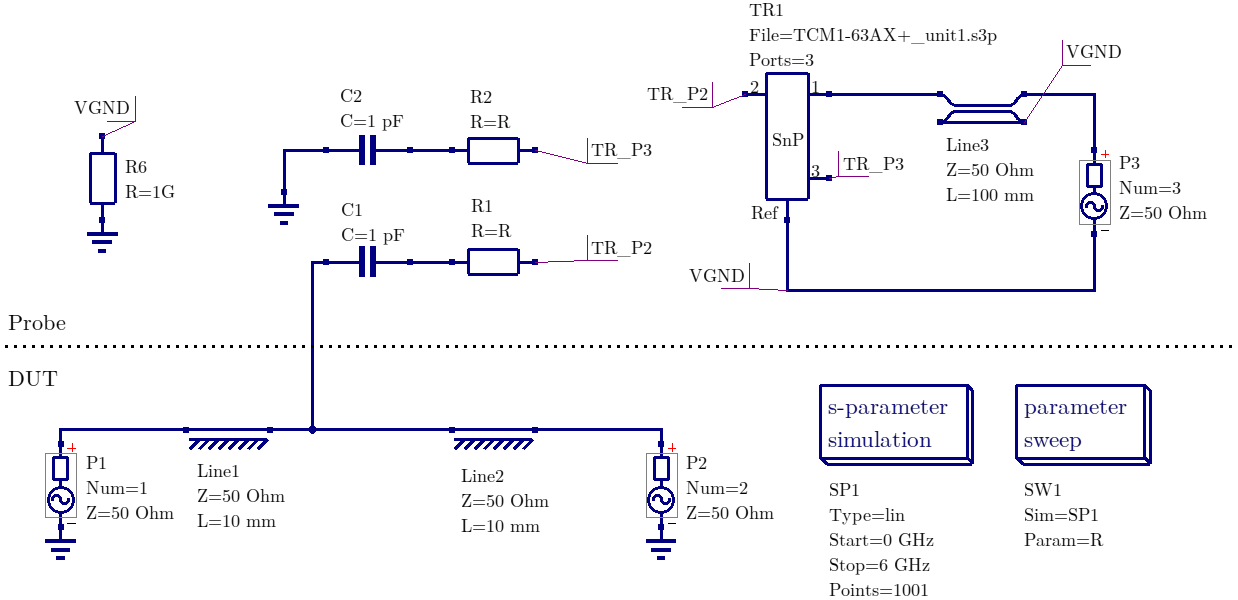The DUT has two ports, P1 and P2, while the output port of the probe is P3.

This simulation model can be used to modify various parameters, especially the values of the two resistors. For instance, the graph below shows a sweep of resistor values for all E6-series values between 100 Ω and 1 kΩ.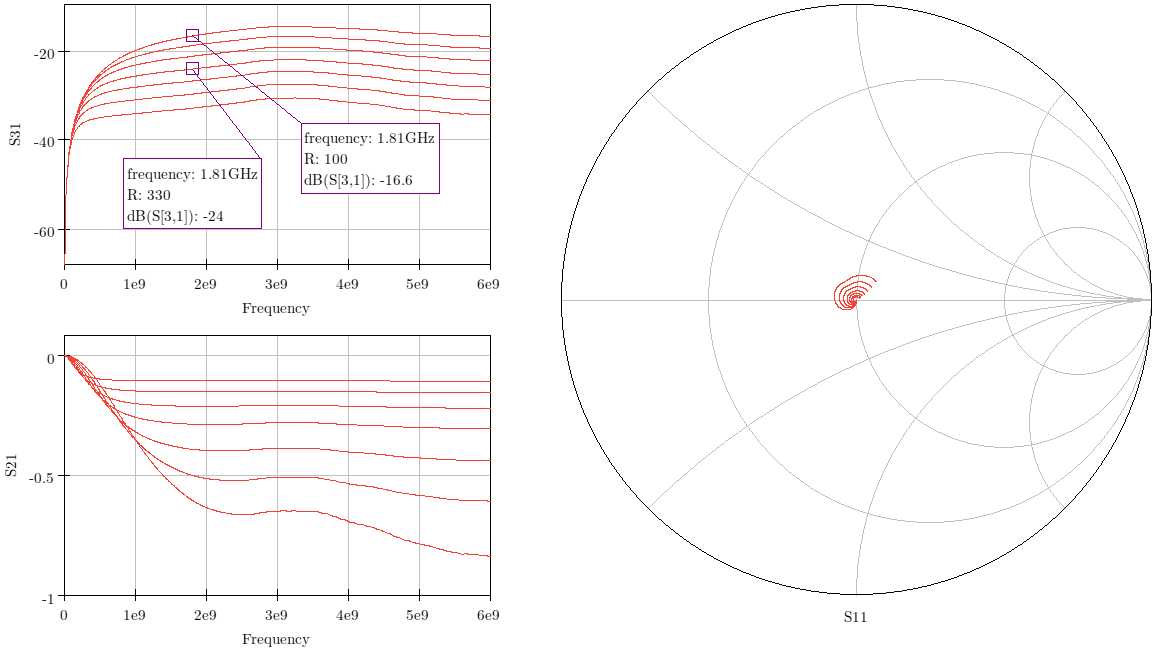I collected the key values from the simulation results in the table below:

R value Probe attenuation (S31) at 2 GHz Effect on DUT (S21) at 2 GHz
100 Ω -16.1 dB -0.6 dB
150 Ω -18.3 dB -0.5 dB
220 Ω -20.8 dB -0.4 dB
330 Ω -23.7 dB -0.3 dB
470 Ω -26.4 dB -0.2 dB
680 Ω -29.3 dB -0.1 dB
1000 Ω -32.5 dB -0.1 dB

### Measurements

Measurements were performed on a test track with two values for the resistors: R=100 Ω (ERJ-PA2F1000X) and R=240 Ω (ERJ-2RKF2400X).

#### Measurements principle

The measurements were performed using a Siglent SVA1032X 3.2 GHz spectrum and vector network analyzer and an example test track (50 Ω microstrip transmission line).

Three metrics were used to evaluate the performance of the probe:

1. Measure the attenuation of the probe (S31 in the simulation).
2. Measure the effect of the probe on the circuit: attenuation due to the probe (S21 in the simulation).
3. Measure the effect of the probe on the circuit: return loss due to the probe (S11 in the simulation).

The VNA is calibrated with short, open, load and through (SOLT) with the test track connected to port 1.

#### Attenuation of the probe

To measure the attenuation of the probe (its transfert function), the test track is terminated with 50 Ω and the power going through the probe is measured on port 2.

The following figure illustrates this setup: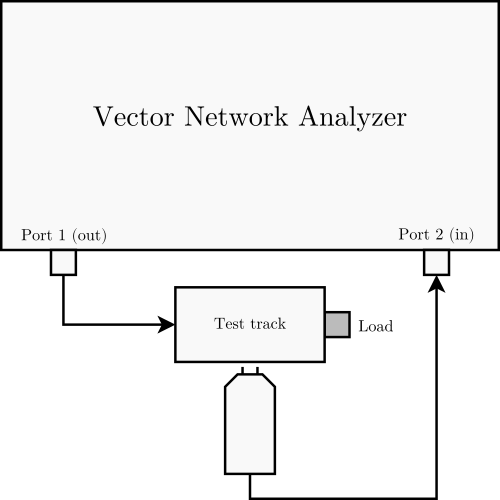Setup #1: measuring the attenuation (transfert function) of the probe.

The following plot shows the probe attenuation measured using setup #1.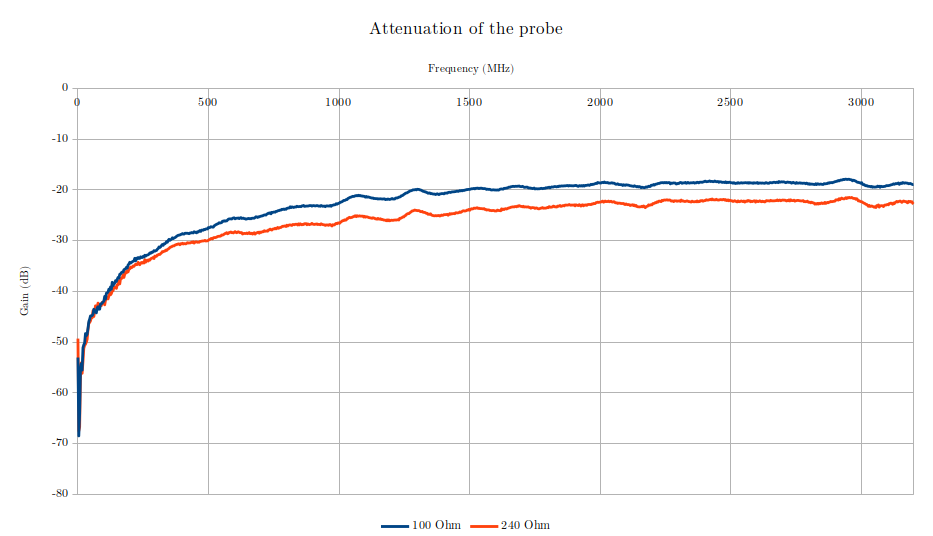Plot of S31 for two values for the resistors.

For this metric, flatter is better. The absolute value characterizes the probe.

#### Loss on the test track due to the probe

To measure the loss on the test track due to the probe, the probe is terminated with 50 Ω and contacting the test track while the power going through the test track is measured on port 2.

The following figure illustrates this setup: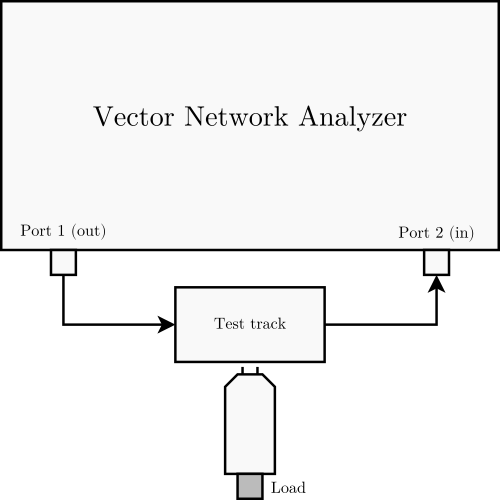Setup #2: measuring the effect of the probe on the circuit.

The following plot shows the test track loss due to the probe (S21) measured using setup #2.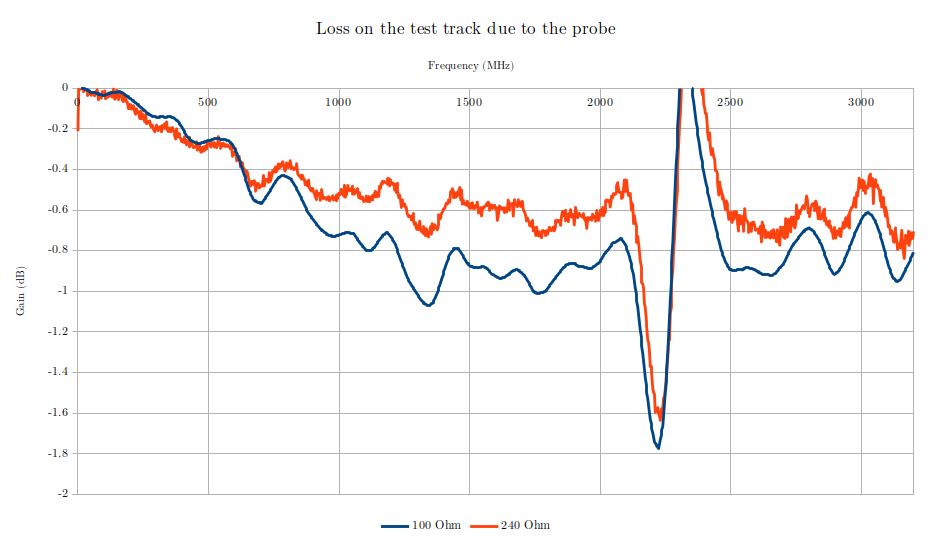Plot of S21 for two values for the resistors.

For this metric, a value closer to 0 dB indicates better performance (less effect on the DUT).

Note: the large oscillation around 2.4 GHz is due to the measurement setup picking up external noise.

#### Return loss on the test track due to the probe

With either setup, S11 can be measured to evaluate the return loss caused by the presence of the probe.

The following plot shows the test track return loss due to the probe (S11) measured using setup #2.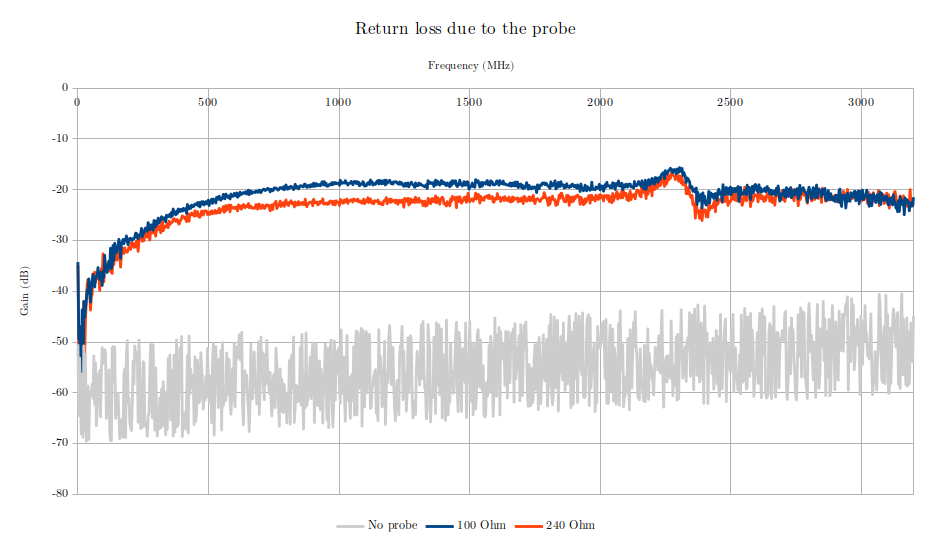Plot of S11 for two values for the resistors.

For this metric, lower values indicate better performance (less return loss).

## Limitations and future improvements

The rev 1.0 of this probe has a limitation in the maximum attenuation it can achieve due to sensitivity to radiated emissions. For the setup used in the measurements detailed above, the noise floor is about -30 dB, meaning that the maximum usable value of the resistors is R=1000 Ω.

The improvements for the rev 2.0 of this probe will include:

• Adding 1 extra ferrite bead for improved handling (lengthen the probe),
• Shortening the path from the pogo pins to the resistors, and
• Developing a lower bandwidth version with an LTCC transformer in addition to the current core and wire version, such as the Mini-Circuits NCS1-332+.

Auteur :